Скачать презентацию Plan for Mon 29 Sept 08 Exam

9c22df716fd4ec3b9e2c44416c16f2ce.ppt

• Количество слайдов: 21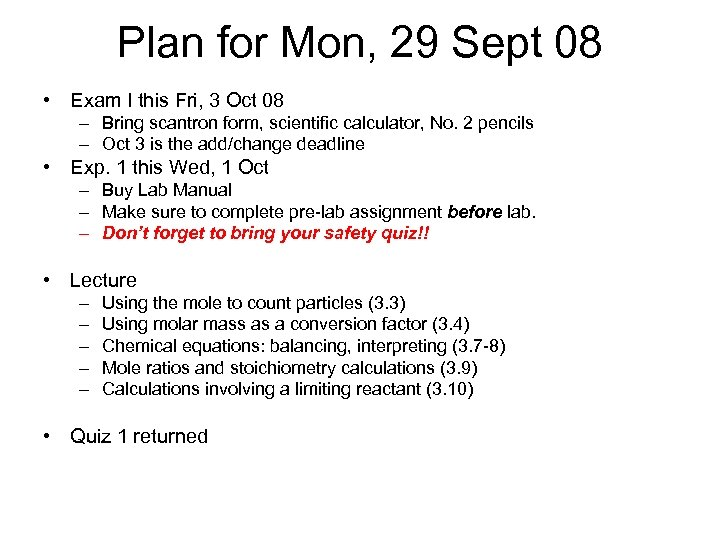Plan for Mon, 29 Sept 08 • Exam I this Fri, 3 Oct 08 – Bring scantron form, scientific calculator, No. 2 pencils – Oct 3 is the add/change deadline • Exp. 1 this Wed, 1 Oct – Buy Lab Manual – Make sure to complete pre-lab assignment before lab. – Don’t forget to bring your safety quiz!! • Lecture – – – Using the mole to count particles (3. 3) Using molar mass as a conversion factor (3. 4) Chemical equations: balancing, interpreting (3. 7 -8) Mole ratios and stoichiometry calculations (3. 9) Calculations involving a limiting reactant (3. 10) • Quiz 1 returned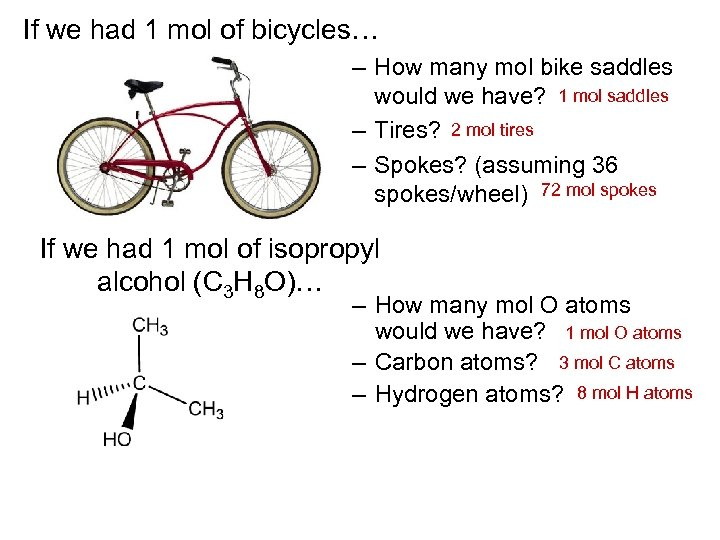If we had 1 mol of bicycles… – How many mol bike saddles would we have? 1 mol saddles – Tires? 2 mol tires – Spokes? (assuming 36 spokes/wheel) 72 mol spokes If we had 1 mol of isopropyl alcohol (C 3 H 8 O)… – How many mol O atoms would we have? 1 mol O atoms – Carbon atoms? 3 mol C atoms – Hydrogen atoms? 8 mol H atoms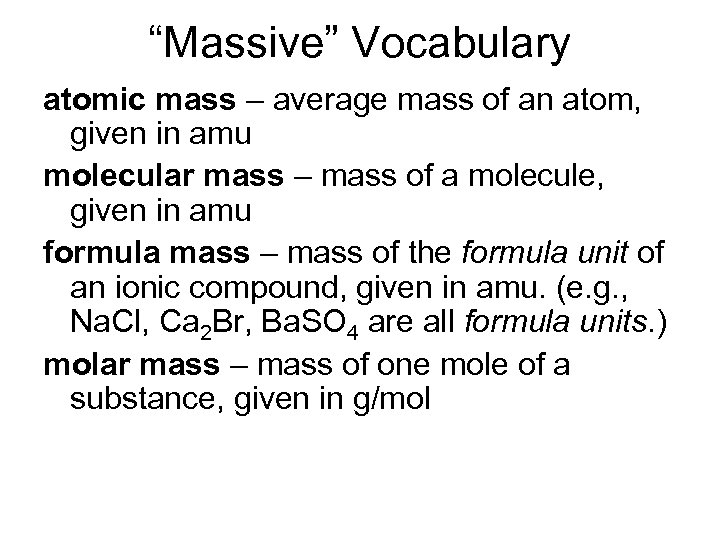“Massive” Vocabulary atomic mass – average mass of an atom, given in amu molecular mass – mass of a molecule, given in amu formula mass – mass of the formula unit of an ionic compound, given in amu. (e. g. , Na. Cl, Ca 2 Br, Ba. SO 4 are all formula units. ) molar mass – mass of one mole of a substance, given in g/mol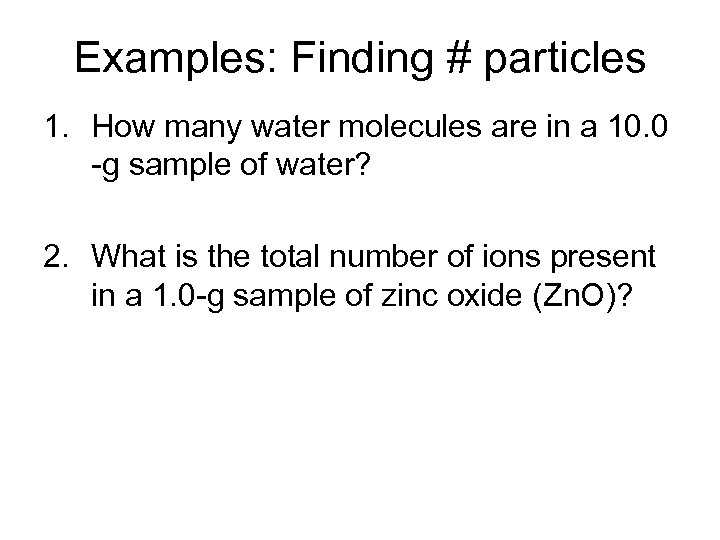Examples: Finding # particles 1. How many water molecules are in a 10. 0 -g sample of water? 2. What is the total number of ions present in a 1. 0 -g sample of zinc oxide (Zn. O)?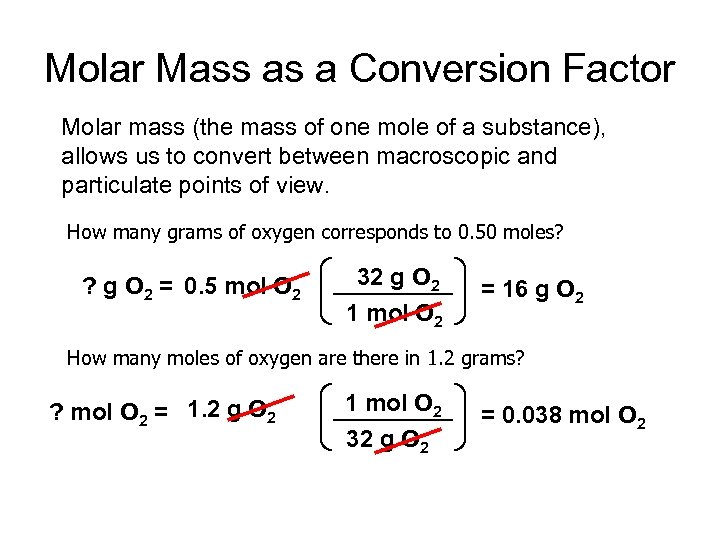Molar Mass as a Conversion Factor Molar mass (the mass of one mole of a substance), allows us to convert between macroscopic and particulate points of view. How many grams of oxygen corresponds to 0. 50 moles? ? g O 2 = 0. 5 mol O 2 32 g O 2 1 mol O 2 = 16 g O 2 How many moles of oxygen are there in 1. 2 grams? ? mol O 2 = 1. 2 g O 2 1 mol O 2 32 g O 2 = 0. 038 mol O 2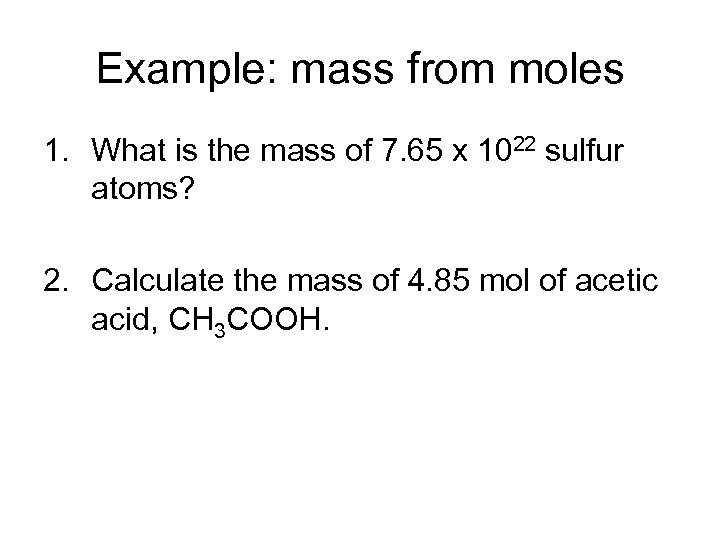Example: mass from moles 1. What is the mass of 7. 65 x 1022 sulfur atoms? 2. Calculate the mass of 4. 85 mol of acetic acid, CH 3 COOH.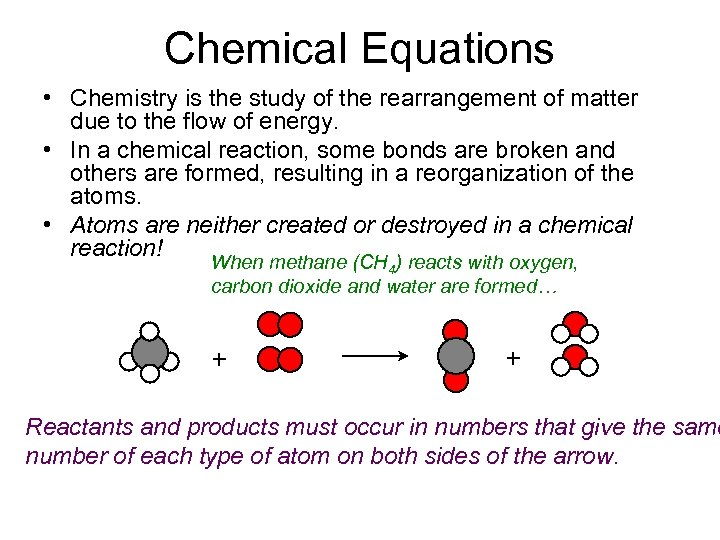Chemical Equations • Chemistry is the study of the rearrangement of matter due to the flow of energy. • In a chemical reaction, some bonds are broken and others are formed, resulting in a reorganization of the atoms. • Atoms are neither created or destroyed in a chemical reaction! When methane (CH 4) reacts with oxygen, carbon dioxide and water are formed… + + Reactants and products must occur in numbers that give the same number of each type of atom on both sides of the arrow.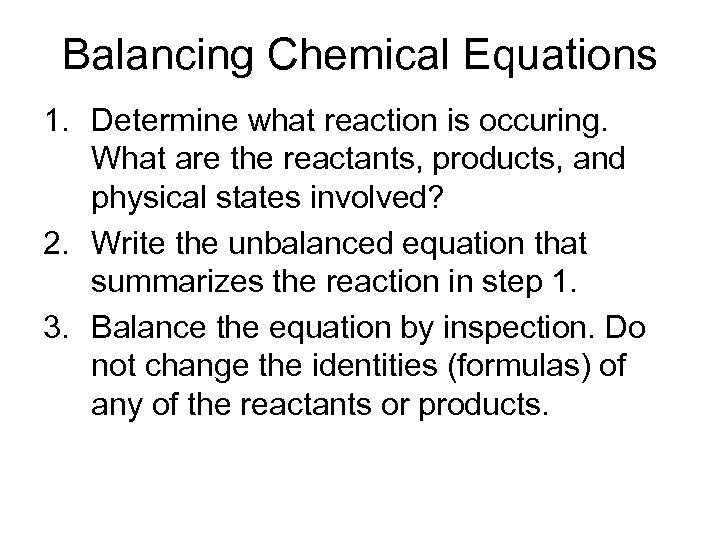Balancing Chemical Equations 1. Determine what reaction is occuring. What are the reactants, products, and physical states involved? 2. Write the unbalanced equation that summarizes the reaction in step 1. 3. Balance the equation by inspection. Do not change the identities (formulas) of any of the reactants or products.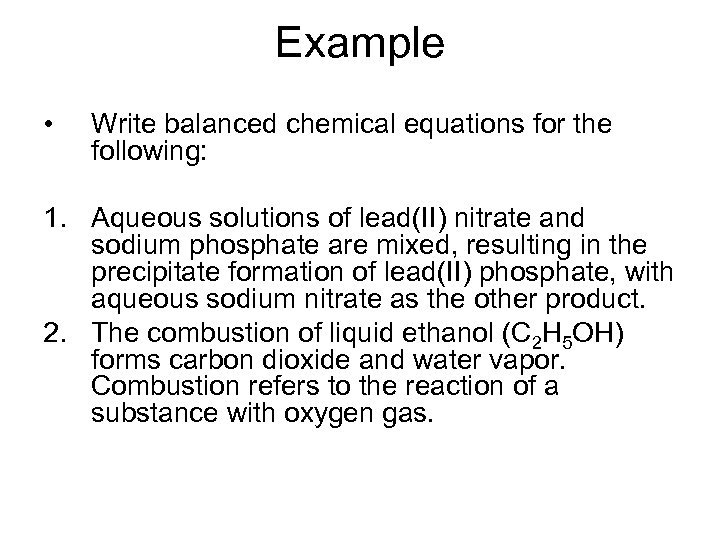Example • Write balanced chemical equations for the following: 1. Aqueous solutions of lead(II) nitrate and sodium phosphate are mixed, resulting in the precipitate formation of lead(II) phosphate, with aqueous sodium nitrate as the other product. 2. The combustion of liquid ethanol (C 2 H 5 OH) forms carbon dioxide and water vapor. Combustion refers to the reaction of a substance with oxygen gas.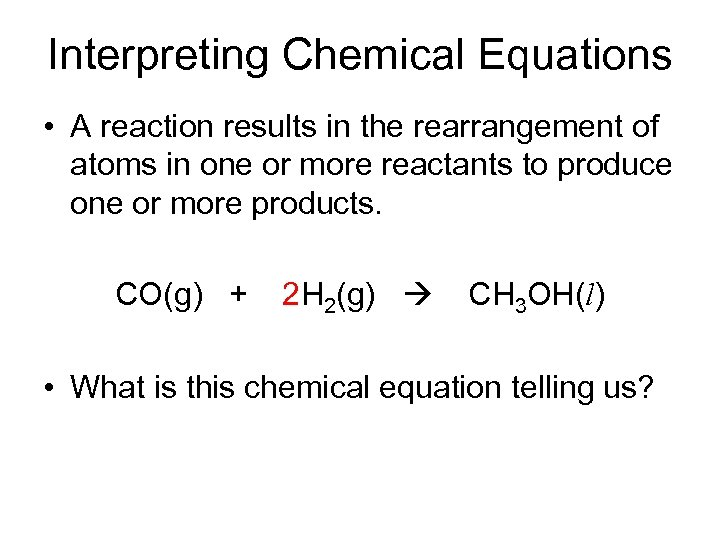Interpreting Chemical Equations • A reaction results in the rearrangement of atoms in one or more reactants to produce one or more products. CO(g) + 2 H 2(g) CH 3 OH(l) • What is this chemical equation telling us?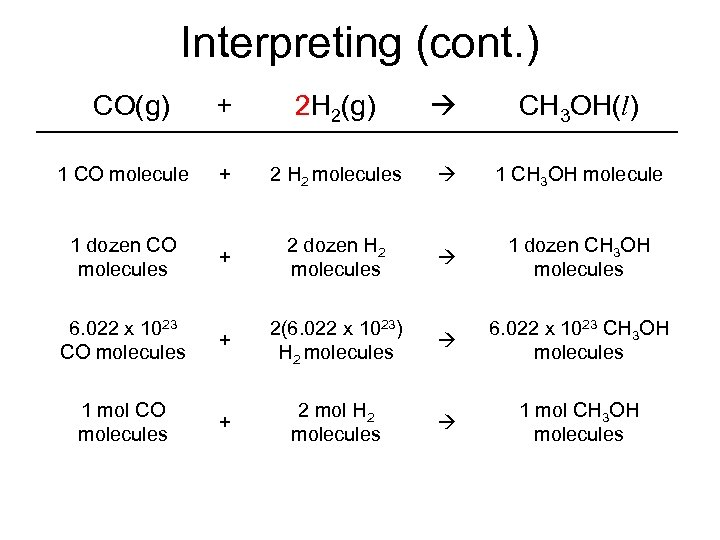Interpreting (cont. ) CO(g) + 2 H 2(g) CH 3 OH(l) 1 CO molecule + 2 H 2 molecules 1 CH 3 OH molecule 1 dozen CO molecules + 2 dozen H 2 molecules 1 dozen CH 3 OH molecules 6. 022 x 1023 CO molecules + 2(6. 022 x 1023) H 2 molecules 6. 022 x 1023 CH 3 OH molecules 1 mol CO molecules + 2 mol H 2 molecules 1 mol CH 3 OH molecules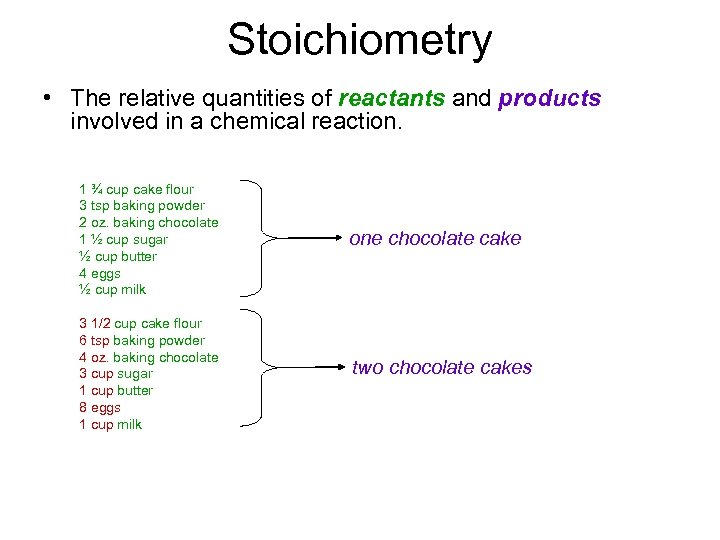Stoichiometry • The relative quantities of reactants and products involved in a chemical reaction. 1 ¾ cup cake flour 3 tsp baking powder 2 oz. baking chocolate 1 ½ cup sugar ½ cup butter 4 eggs ½ cup milk one chocolate cake 3 1/2 cup cake flour 6 tsp baking powder 4 oz. baking chocolate 3 cup sugar 1 cup butter 8 eggs 1 cup milk two chocolate cakes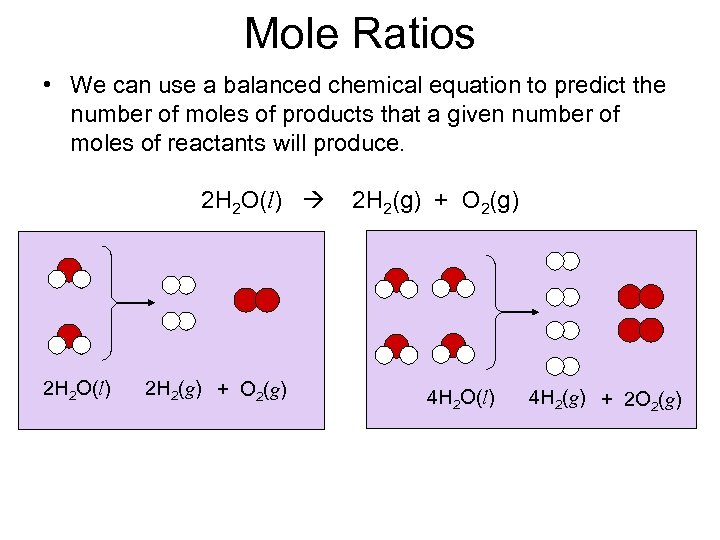Mole Ratios • We can use a balanced chemical equation to predict the number of moles of products that a given number of moles of reactants will produce. 2 H 2 O(l) 2 H 2(g) + O 2(g) 4 H 2 O(l) 4 H 2(g) + 2 O 2(g)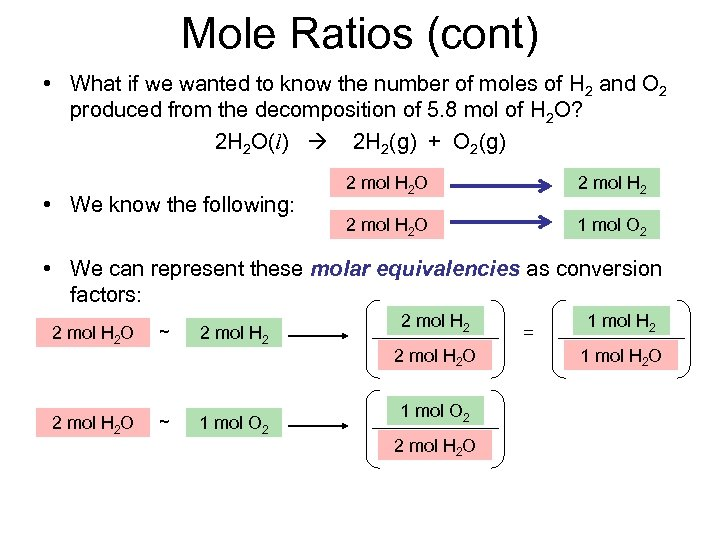Mole Ratios (cont) • What if we wanted to know the number of moles of H 2 and O 2 produced from the decomposition of 5. 8 mol of H 2 O? 2 H 2 O(l) 2 H 2(g) + O 2(g) • We know the following: 2 mol H 2 O 1 mol O 2 • We can represent these molar equivalencies as conversion factors: 2 mol H 2 O ~ 2 mol H 2 1 mol O 2 2 mol H 2 O = 1 mol H 2 O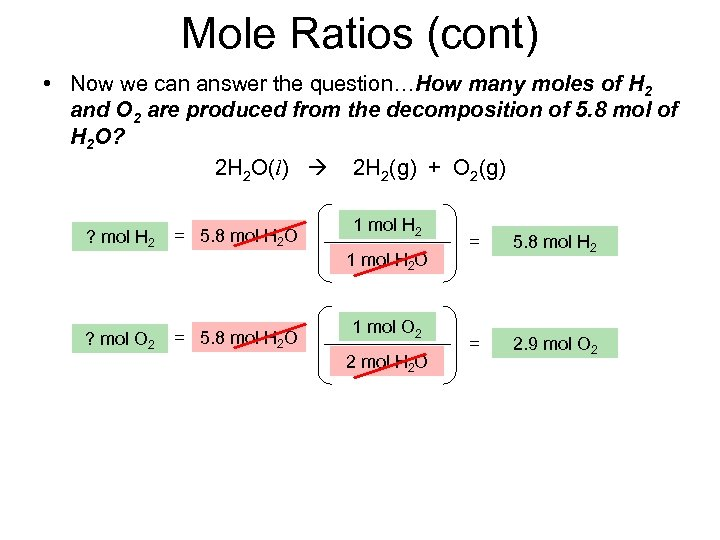Mole Ratios (cont) • Now we can answer the question…How many moles of H 2 and O 2 are produced from the decomposition of 5. 8 mol of H 2 O? 2 H 2 O(l) 2 H 2(g) + O 2(g) ? mol H 2 = 5. 8 mol H 2 O ? mol O 2 = 5. 8 mol H 2 O 1 mol O 2 2 mol H 2 O = 5. 8 mol H 2 = 2. 9 mol O 2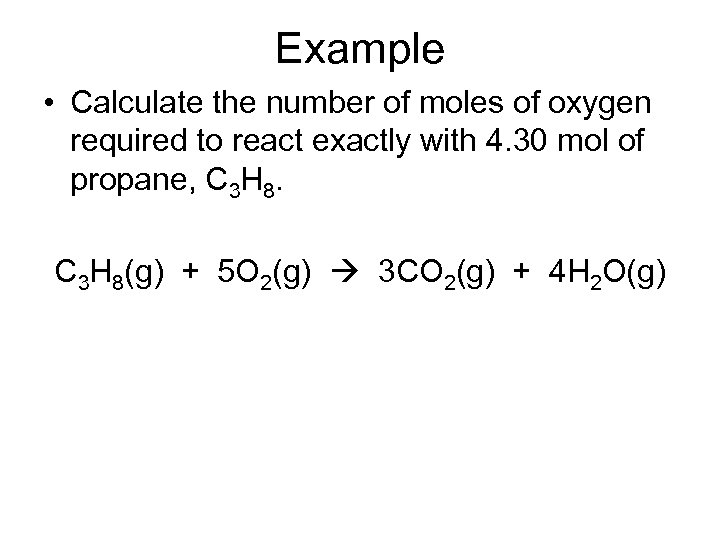Example • Calculate the number of moles of oxygen required to react exactly with 4. 30 mol of propane, C 3 H 8(g) + 5 O 2(g) 3 CO 2(g) + 4 H 2 O(g)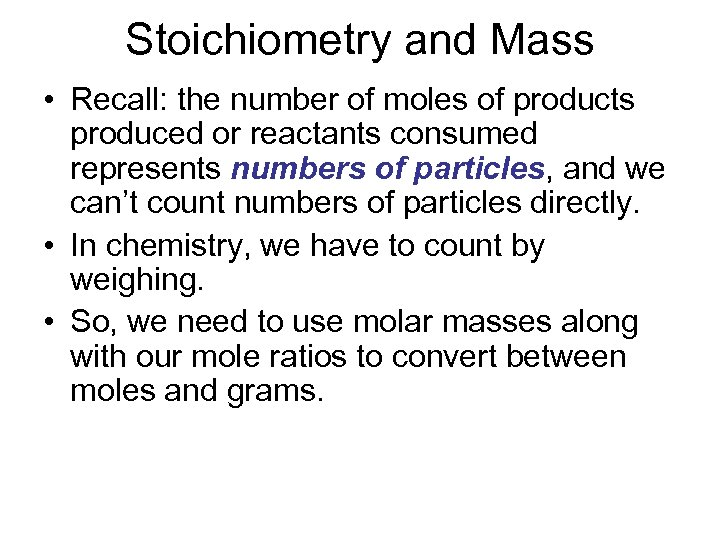Stoichiometry and Mass • Recall: the number of moles of products produced or reactants consumed represents numbers of particles, and we can’t count numbers of particles directly. • In chemistry, we have to count by weighing. • So, we need to use molar masses along with our mole ratios to convert between moles and grams.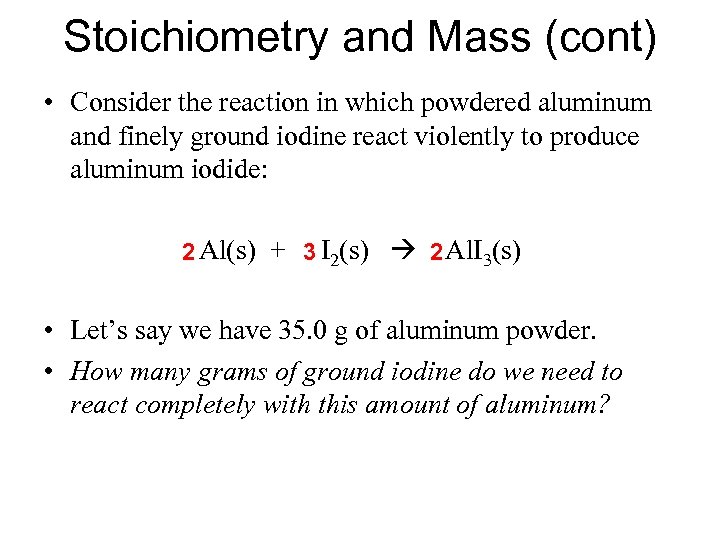Stoichiometry and Mass (cont) • Consider the reaction in which powdered aluminum and finely ground iodine react violently to produce aluminum iodide: 2 Al(s) + 3 I 2(s) 2 Al. I 3(s) • Let’s say we have 35. 0 g of aluminum powder. • How many grams of ground iodine do we need to react completely with this amount of aluminum?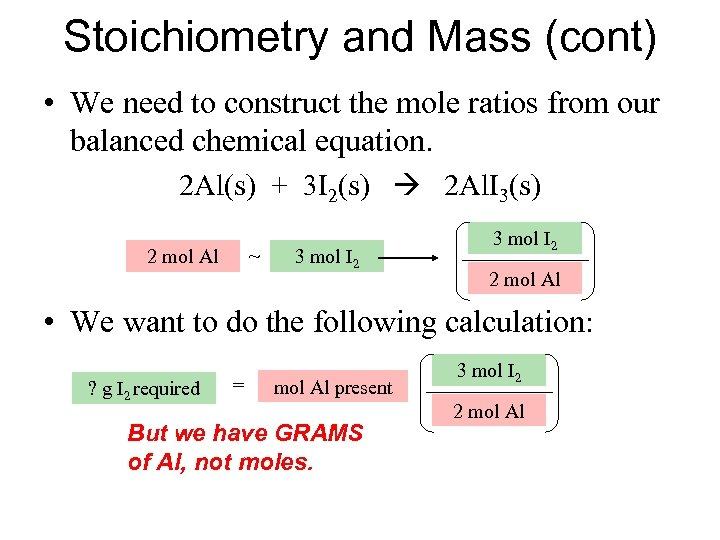Stoichiometry and Mass (cont) • We need to construct the mole ratios from our balanced chemical equation. 2 Al(s) + 3 I 2(s) 2 Al. I 3(s) ~ 2 mol Al 3 mol I 2 2 mol Al • We want to do the following calculation: ? g I 2 required = mol Al present But we have GRAMS of Al, not moles. 3 mol I 2 2 mol Al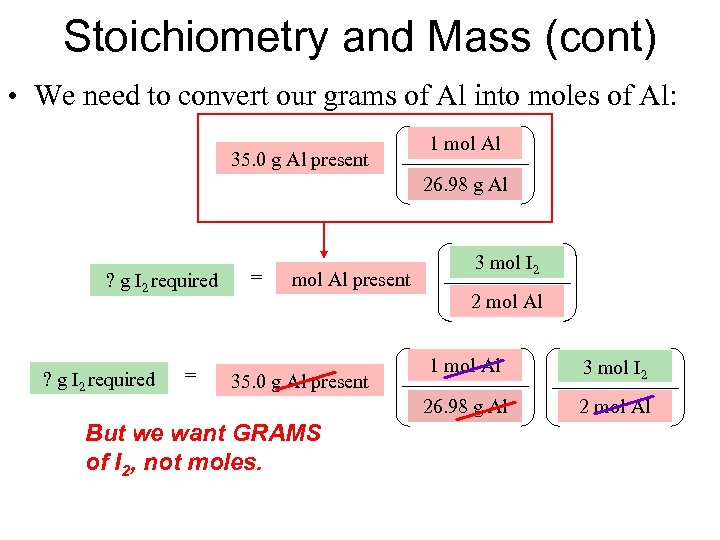Stoichiometry and Mass (cont) • We need to convert our grams of Al into moles of Al: 35. 0 g Al present 1 mol Al 26. 98 g Al ? g I 2 required = mol Al present 3 mol I 2 2 mol Al = 35. 0 g Al present But we want GRAMS of I 2, not moles. 1 mol Al 3 mol I 2 26. 98 g Al ? g I 2 required 2 mol Al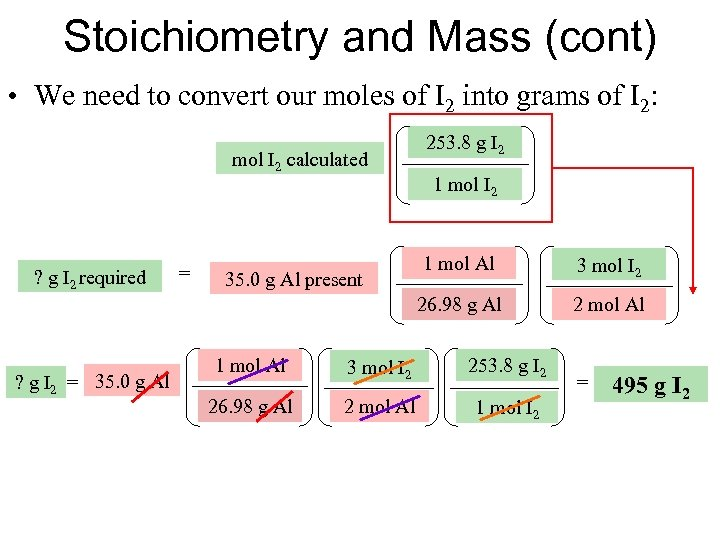Stoichiometry and Mass (cont) • We need to convert our moles of I 2 into grams of I 2: mol I 2 calculated 253. 8 g I 2 1 mol I 2 ? g I 2 = 35. 0 g Al present 1 mol Al 3 mol I 2 26. 98 g Al ? g I 2 required 2 mol Al 1 mol Al 3 mol I 2 253. 8 g I 2 26. 98 g Al 2 mol Al 1 mol I 2 = 495 g I 2